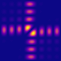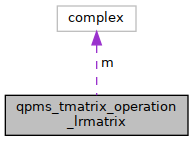QPMS Electromagnetic multiple scattering library and toolkit.
qpms_tmatrix_operation_lrmatrix Struct Reference

General matrix transformation. More...

#include <tmatrices.h>

Collaboration diagram for qpms_tmatrix_operation_lrmatrix:[legend]

## Data Fields

complex double * m
Raw matrix data of M in row-major order. More...

size_t m_size
Total size of m matrix in terms of sizeof(complex double).

bool owns_m
Whether m is owned by this;.

## Detailed Description

General matrix transformation.

$T' = MTM^\dagger$

spec for qpms_tmatrix_operation_t.

## ◆ m

 complex double* qpms_tmatrix_operation_lrmatrix::m

Raw matrix data of M in row-major order.

The matrix must be taylored for the given bspec!

The documentation for this struct was generated from the following file: